# Parameter-introduction method

A method in which the right-hand side of a system of differential equations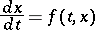(1)

is represented in the form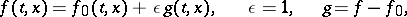where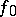is the principal part (in some sense) of the vector function, andis the totality of second-order terms. The decomposition ofintoandis usually determined by the physical or analytical nature of the problem described by the system (1). Besides this system, the system with a parameter,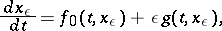(2)

is also considered; if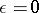, this system becomes the degenerate system(3)

If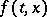and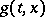are holomorphic in a neighbourhood of a point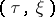, the system (2) has the solution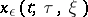,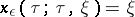for values ofwhich are, in modulus, sufficiently small. This solution can be represented in a neighbourhood of the initial valuesas a power series in: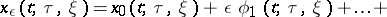(4)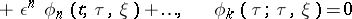(in certain cases non-zero initial values may also be specified for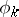). If the series (4) converges for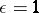, it supplies the solution of the system (1) with initial values. For an effective construction of the coefficients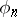it is sufficient to have the general solution of system (3) and a partial solution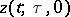of an arbitrary system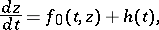whereis holomorphic in a neighbourhood of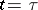.

In particular, allcan be successively determined by quadratures if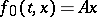, whereis a constant matrix.

The method of parameter introduction is very extensively employed in the theory of non-linear oscillations  for the construction of periodic solutions of the system (1). (See also Small parameter, method of the.) The method was employed by P. Painlevé to classify second-order differential equations whose solutions have no moving critical singular points (cf. Painlevé equation). The following theorem is true: Systems with fixed critical points can only be constituted by systems (1) which, after the introduction of a suitable parameter, have systems without moving critical singular points as the degenerate systems (3). The parameter-introduction method is widely employed to construct new classes of essentially non-linear differential systems (1) without moving critical singular points, and in the study of systems belonging to these classes (cf. Singular point of a differential equation).